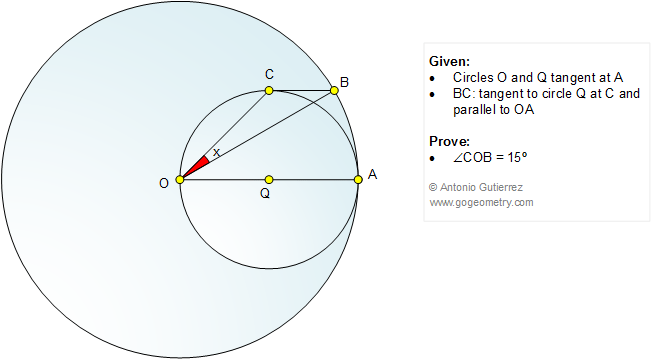Problem 1087: Tangent Circles, Parallel, 15 Degrees, Angle Level: High School, College, Math Education The figure below shows the circles of centers O and Q tangent at A. BC is tangent to circle Q at C and parallel to OA. Prove that angle COB = 15 degrees.Recent Additions< PREVIOUS PROBLEM  |  NEXT PROBLEM >